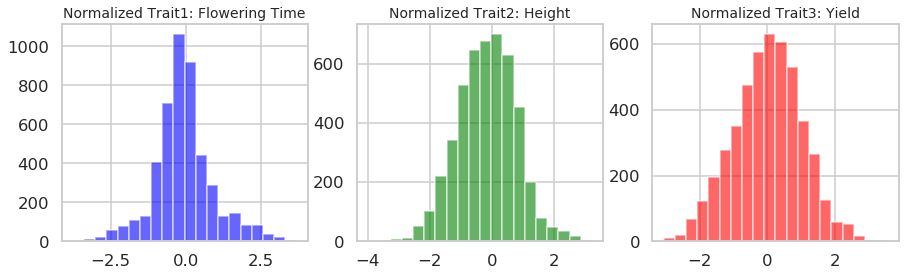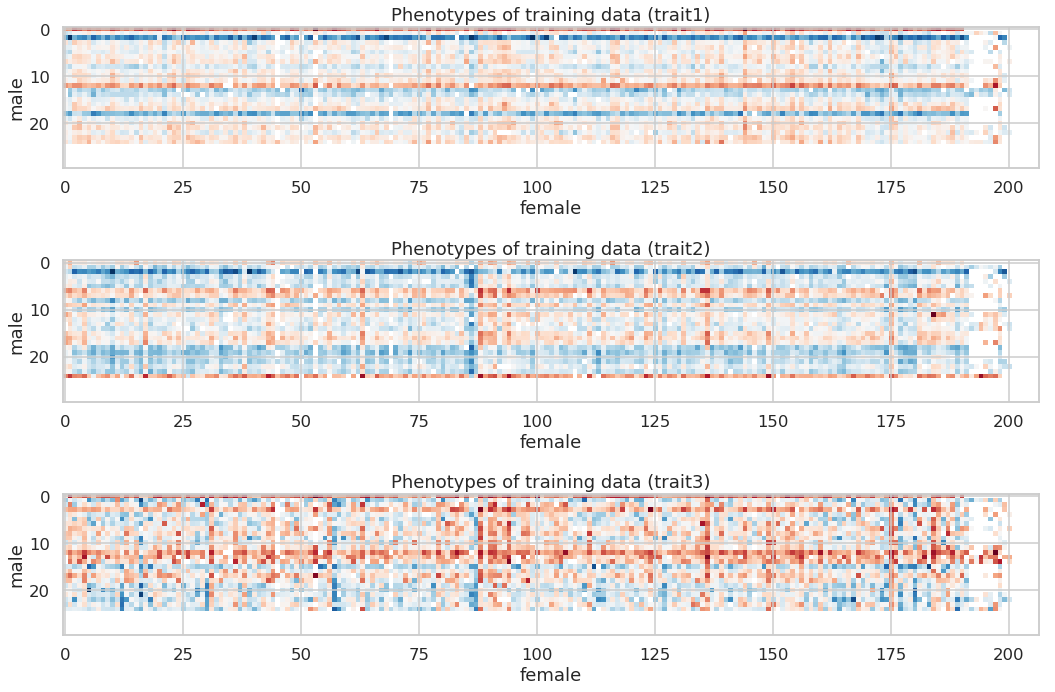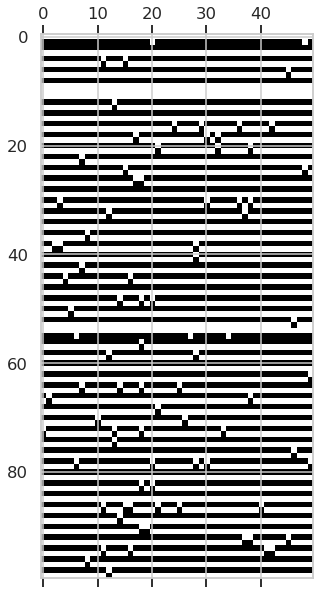# A. eMaize

## eMaize背景简介

eMaize挑战赛是一个通过机器学习方法预测玉米性状的比赛，要求我们以SNP作为特征，通过训练一个模型，对玉米的三个性状进行预测

• 介绍数据的情况，使用方式
• 具体任务要求
• 一些机器学习的概念方法以及工具使用

## 编程工具介绍

pip install tqdm
conda install sklearn

#导入必需的库
%pylab inline
import numpy as np
from sklearn.random_projection import SparseRandomProjection
import scipy.stats
import pandas as pd
import sklearn
import h5py
from sklearn.metrics import r2_score
from scipy.stats.stats import pearsonr
from sklearn.linear_model import LinearRegression
import xgboost
from xgboost.sklearn import XGBRegressor
from sklearn.linear_model import Ridge
from sklearn.kernel_ridge import KernelRidge
from sklearn import neighbors
from sklearn.ensemble import RandomForestRegressor
from sklearn.gaussian_process import GaussianProcessRegressor
from sklearn.gaussian_process.kernels import DotProduct
from tqdm import tqdm_notebook as tqdm
from IPython.display import display, Image
import seaborn as sns
import warnings
warnings.filterwarnings('ignore')

styles = ["white","dark",'whitegrid',"darkgrid"]
contexts = ['paper','talk','poster','notebook']
sns.set_context(contexts)
sns.set_style(styles)


## 数据介绍

• genotype：SNP (Single-nucleotide polymorphism) 数据

每个位点可能有三种情况，如AA，AT，TT，每个样本SNP位点约为190万个，数据位置data/genotype_2bit/，包含十个染色体各自的SNP，使用时需要整合在一起。

• trait

共三种，trait1开花期，trait2株高，trait3产量，为连续值，只提供训练集样本的性状数据。data/pheno_emaize.txt

### Genotype数据

#### SNP数据存储格式

txt存储格式不适合大数据读取的问题，对内存的占用过多

tips: 在命令行查看数据shape的方法为： cd至文件路径下，输入：h5ls filename

#使用h5py读取5000个SNP，此时数据为原始的碱基信息
with h5py.File('data/snp_5000','r') as f:
print (list(f.keys()))
snps = f['snp'][:]

#使用h5py读取5000个SNP，此时为原始的碱基信息转化为数值信息后的数据
with h5py.File('data/2bit_geno','r') as f:
print (list(f.keys()))
snps_2bit = f['data'][:]
snps_2bit.shape

#使用h5py读取一个染色体的SNP示例，此时文件内为我们处理好的数值化数据，注意需要先解压文件：
with h5py.File('data/genotype_2bit/chr1.h5','r') as f:
print (list(f.keys()))
chr1_snps = f['data'][:]
chr1_snps.shape, chr1_snps.dtype


#### 性状数据

traits = pd.read_csv('data/pheno_emaize.txt','\t')
display(traits.tail())


trait1 = np.array(traits['trait1'])[:4754]
trait2 = np.array(traits['trait2'])[:4754]
trait3 = np.array(traits['trait3'])[:4754]
fig, ax = plt.subplots(1,3, figsize=(15,4))
ax.hist(trait1,bins = 20,color='b',alpha=0.6)
ax.set_title('Normalized Trait1: Flowering Time',fontsize=14)
ax.hist(trait2,bins = 20,color='g',alpha=0.6)
ax.set_title('Normalized Trait2: Height',fontsize=14)
ax.hist(trait3,bins = 20,color='r',alpha=0.6)
ax.set_title('Normalized Trait3: Yield',fontsize=14)## Quiz具体要求

• 完成特征选择和特征筛除工作
• 完成对三种性状的预测并提交预测结果，允许多次提交预测结果以获得更好的结果。
• 提交一份工作报告，中英文不限，同时提交源代码
• 选择性完成加分项内容。

### 特征选择和冗余特征筛除

• 特征选择：
• ANOVA（方差分析）
• 基于模型权重排序（如线性模型）
• 降维：
• PCA
• SVD
• Random Projection

tips: 请思考是否需要针对特征选择或者降维后的数据做scale

### 完成对测试集三种性状的预测，尝试得到尽可能好的预测结果

• 请思考和探索使用何种回归模型，读者可以尝试多种模型并比较其结果，在编程工具介绍部分读者可以看到一些机器学习模型的方法，也推荐读者思考和使用其他模型。
• 思考和探索是否对每个性状使用不同的模型
• 根据训练集与测试集的特殊划分方式（见查看训练集与测试集的划分部分）思考可以使用的策略。

### 加分内容

#### 对模型进行鲁棒性测试 (10')

• 在读者已有的数据（训练集数据）上进行多轮（如100、1000轮）交叉验证，测试模型的鲁棒性
• 设计不同的数据集划分方式，除了随机划分训练集与验证集，还可以有其他特殊的设计方法
• 统计测试结果，以多种形式展现，包括统计数据和图示。

#### 混合线性模型 (20')

• 可以研究并调用FaST-LMM package，研究其原理并应用于我们的数据中，试图在测试集上获得好的预测结果(5')
• 根据其思路进行改进，设计一个类似的混合线性模型，并且可以通过快速的交叉验证挑选超参数，最终在测试集上获得好的效果。详细内容可以参考这篇文档。(15')

## 补充知识（选读）

### 测试预测结果

def generate_parent_table(phenotype_file):
pedigree = phenotypes['pedigree'].str.split('_', expand=True)
pedigree.columns = ['f', 'X', 'm']
phenotypes = pd.concat([phenotypes, pedigree], axis=1)
phenotypes['number'] = np.arange(phenotypes.shape)
parent_table = phenotypes.pivot_table(values='number'
, index=['m'], columns=['f'], dropna=False)
male_ids = ['m%d' % i for i in range(1, parent_table.shape + 1)]
female_ids = ['f%d' % i for i in range(1, parent_table.shape + 1)]
parent_table = parent_table.loc[male_ids, female_ids]
return parent_table
phenotype_file = 'data/pheno_emaize.txt'
parent_table = generate_parent_table(phenotype_file)
fig, ax = subplots(3,1, figsize=(15, 10))
for i in range(3):
trait = ['trait1','trait2','trait3'][i]
ax[i].imshow(np.take(np.ravel(phenotypes[trait].values), parent_table), cmap=cm.RdBu)
ax[i].set_title('Phenotypes of training data (%s)'%trait)
ax[i].set_ylabel('male')
ax[i].set_xlabel('female')
plt.tight_layout()fig,ax=plt.subplots(figsize=(20,5))
testexample = np.zeros([30,207])
testexample[25:,:202] = 100
testexample[:25,202:] = 200
testexample[25:,202:] = 300
ax.imshow(testexample,cmap='jet')$r^2 = 1-\frac{SS_{res}}{SS_{tot}}$
$PCC = \frac{cov(X,Y)}{\sigma_X \sigma_Y} = \frac{E[(X-\mu_X)(Y-\mu_Y)]}{\sigma_X \sigma_Y}$

### 将SNP数据编码为向量

• 转化为0、1、2。找到minor allele frequency（MAF），即两种碱基（如A、T）中出现频率低的那个，以A作为MAF为例，则TT为0，AT为1，AA为2，这样可以突出MAF
• 转化为3-bit one hot vector,$[1,0,0]^T,[0,1,0]^T,[0,0,1]^T$这样可以保持三种向量在空间距离的一致
• 转化为2-bit vector,则AA，AT，TT分别编为$[1,0]^T,[1,1]^T,[0,1]^T$,不需要考虑MAF

我们采取第三种方式处理了数据并提供给读者

#### 具体处理过程

def convert_2bit(seq):
genotypes = np.zeros([6210,2])
a = seq.split('/')
for i in range(6210):
if seq[4:][i] == a + a:
genotypes[i] = np.array([0,1])
if seq[4:][i] == a + a:
genotypes[i] = np.array([1,0])
if seq[4:][i] == a + a:
genotypes[i] = np.array([1,1])
genotypes = genotypes.astype('int').T
return genotypes


snps = snps.astype('str')
snps.shape

convert_2bit(snps.astype('str'))

geno_conv = np.ndarray([10000,6210])
for i in tqdm(range(5000)):
geno_conv[i*2:(i+1)*2] = convert_2bit(snps[i+1])

#查看SNP的大致情况，取前100行（50个位点），前50个样本，
fig, ax = plt.subplots(figsize=(20,10))
ax.matshow(geno_conv[:100,:50],cmap = cm.binary_r)### 模型鲁棒性测试

#### 设计特殊的交叉验证方式

• random
• by female
• by male
• cross sampling
for method in ('random', 'by_female', 'by_male', 'cross'):
with h5py.File('data/cv_index.%s'%method, 'r') as f:
index_train = f['0/train'][:]
index_test = f['0/test'][:]
fig, ax = subplots(2, 1, figsize=(16, 6))
sampling_table = np.zeros(np.prod(parent_table.shape))
sampling_table[index_train] = 1
sampling_table = np.take(sampling_table, parent_table)
ax.imshow(sampling_table, cmap=cm.Greys)
ax.set_title('Training samples (%s)'%method)

sampling_table = np.zeros(np.prod(parent_table.shape))
sampling_table[index_test] = 1
sampling_table = np.take(sampling_table, parent_table)
ax.imshow(sampling_table, cmap=cm.Greys)
ax.set_title('Test samples (%s)'%method)
plt.tight_layout()


### ANOVA加速算法